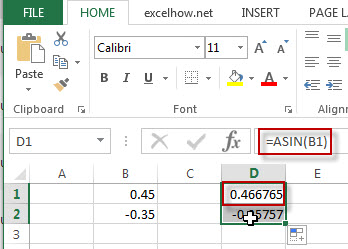## Excel ASIN Function

This post will guide you how to use Excel ASIN function with syntax and examples in Microsoft excel.

### Description

The Excel ASIN function returns the arcsine value of a number.

The ASIN function is a build-in function in Microsoft Excel and it is categorized as a Math and Trigonometry Function.

The ASIN function is available in Excel 2016, Excel 2013, Excel 2010, Excel 2007, Excel 2003, Excel XP, Excel 2000, Excel 2011 for Mac.

### Syntax

The syntax of the ASIN function is as below:

= ASIN (number)

Where the ASIN function argument is:
Number – This is a required argument.  A number between -1 and 1 that you want the arccosine value.

### Example

The below examples will show you how to use Excel ASIN function to return the arcsine value of a number.

#1 = ASIN (B1)Note: the above formula will return the arcsine value of B1 cell.